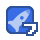### Numerical Python Scientific Computing and Data Science Applications, 2nd Edition

4星（超过85%的资源）86收藏

Numerical Python: Scientific Computing and Data Science Applications with Numpy, SciPy and Matplotlib By 作者: Robert Johansson ISBN-10 书号: 1484242459 ISBN-13 书号: 9781484242452 Edition 版本: 2nd ed. 出版日期: 2018-12-25 pages 页数: (700 ) Work with vectors and matrices using NumPy Plot and visualize data with Matplotlib Perform data analysis tasks with Pandas and SciPy Review statistical modeling and machine learning with statsmodels and scikit-learn Optimize Python code using Numba and Cython Cover Front Matter 1. Introduction to Computing with Python 2. Vectors, Matrices, and Multidimensional Arrays 3. Symbolic Computing 4. Plotting and Visualization 5. Equation Solving 6. Optimization 7. Interpolation 8. Integration 9. Ordinary Differential Equations 10. Sparse Matrices and Graphs 11. Partial Differential Equations 12. Data Processing and Analysis 13. Statistics 14. Statistical Modeling 15. Machine Learning 16. Bayesian Statistics 17. Sinal Processing 18. Data Input and Output 19. Code Optimization

...展开详情

2021-03-05

2019-12-19

2019-12-10

• 392
资源
• 20
粉丝
•等级Numerical Python Scientific Computing and Data Science Applications, 2nd Edition 50积分/C币 立即下载
1/12750积分/C币 立即下载 ＞